# Bayesian Learning Algorithm What is Bayesian Algorithm n

• Slides: 13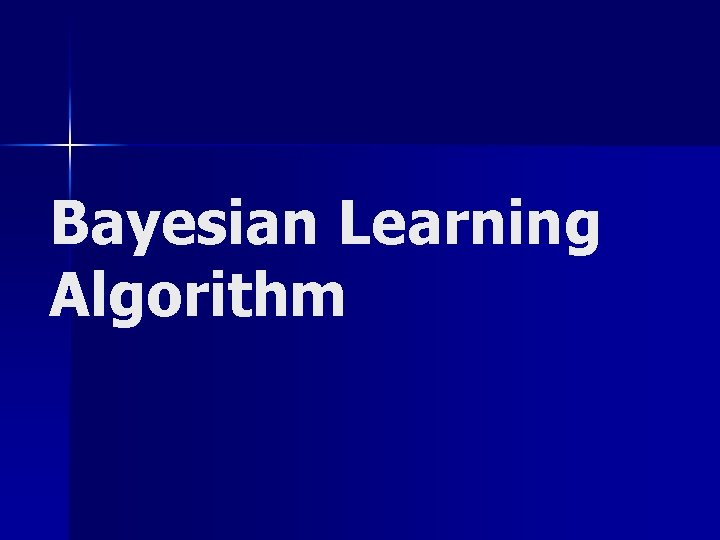Bayesian Learning AlgorithmWhat is Bayesian Algorithm? n Bayesian learning algorithm is a method of calculating probabilities for hypothesis n One of the most practical approaches to certain type of learning problemsUse of Bayesian analysis n Used to justify a design choice in neural network algorithm n Provides perspective for understanding other learning algorithms n Outperforms other methods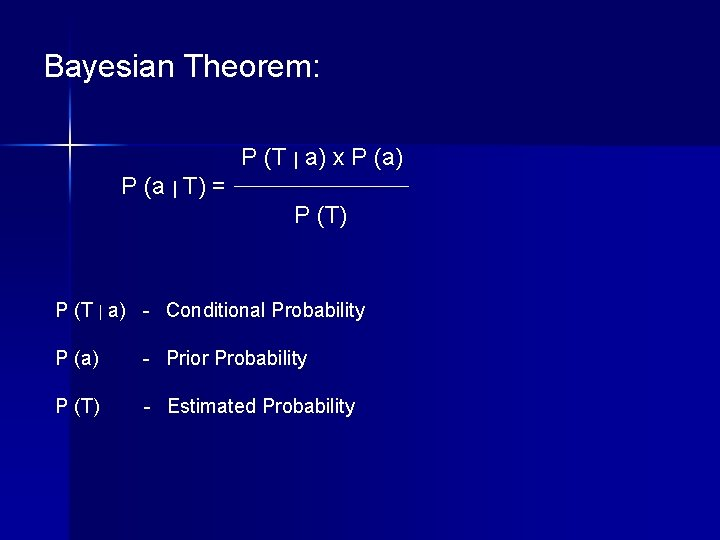Bayesian Theorem: P (T | a) x P (a) P (a | T) = P (T) P (T | a) - Conditional Probability P (a) - Prior Probability P (T) - Estimated Probability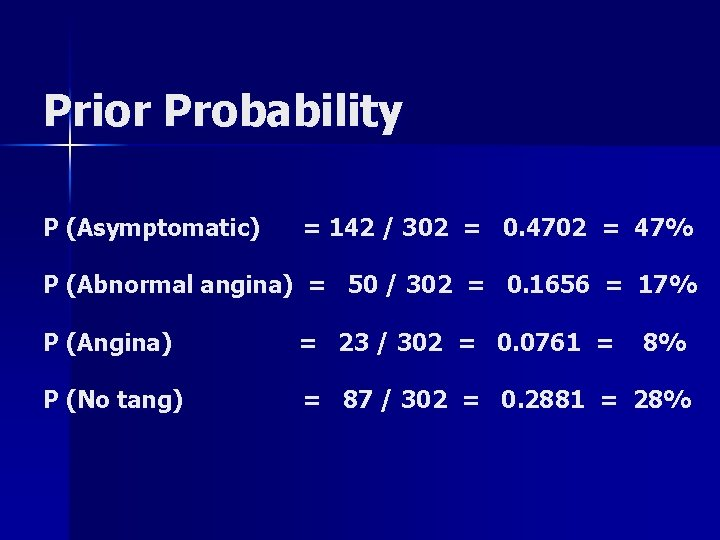Prior Probability P (Asymptomatic) = 142 / 302 = 0. 4702 = 47% P (Abnormal angina) = 50 / 302 = 0. 1656 = 17% P (Angina) = 23 / 302 = 0. 0761 = 8% P (No tang) = 87 / 302 = 0. 2881 = 28%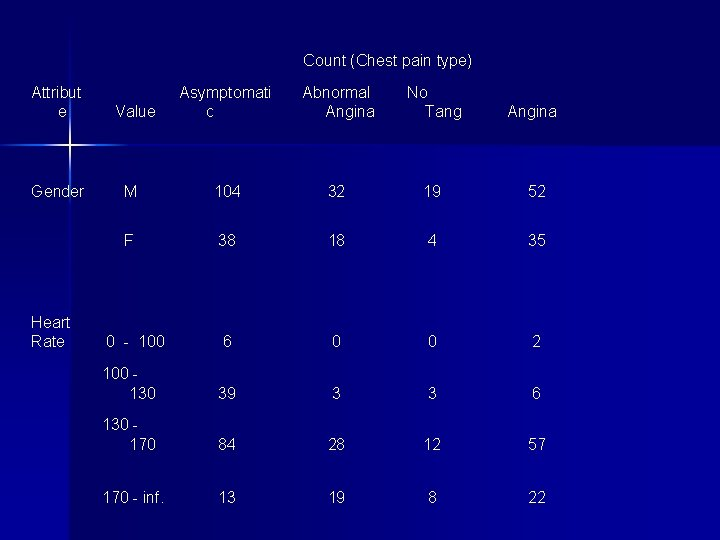Count (Chest pain type) Attribut e Gender Heart Rate Asymptomati c Abnormal Angina No Tang Angina M 104 32 19 52 F 38 18 4 35 0 - 100 6 0 0 2 100 130 39 3 3 6 130 170 84 28 12 57 170 - inf. 13 19 8 22 Value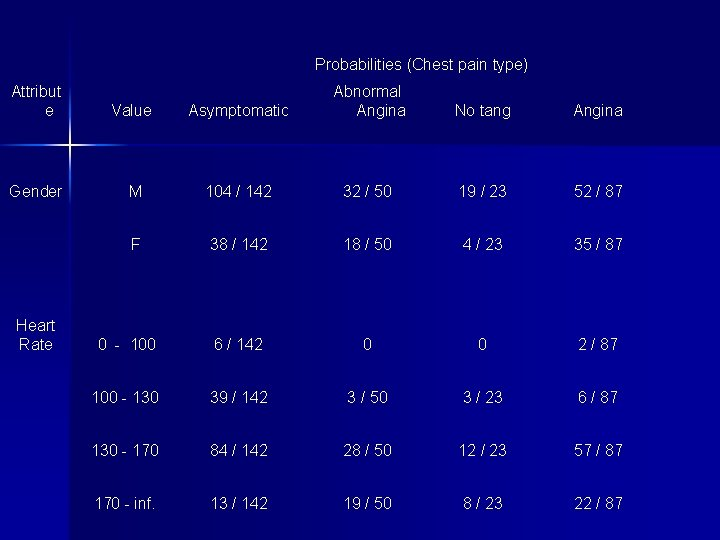Probabilities (Chest pain type) Attribut e Value Gender Heart Rate Asymptomatic Abnormal Angina No tang Angina M 104 / 142 32 / 50 19 / 23 52 / 87 F 38 / 142 18 / 50 4 / 23 35 / 87 0 - 100 6 / 142 0 0 2 / 87 100 - 130 39 / 142 3 / 50 3 / 23 6 / 87 130 - 170 84 / 142 28 / 50 12 / 23 57 / 87 170 - inf. 13 / 142 19 / 50 8 / 23 22 / 87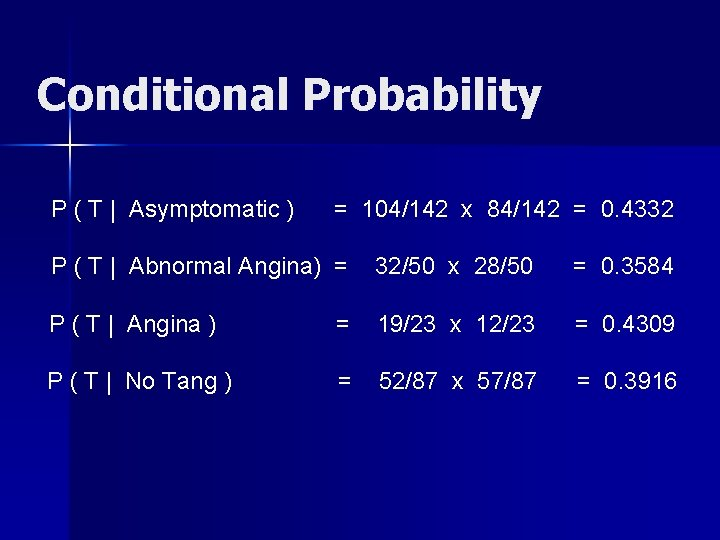Conditional Probability P ( T | Asymptomatic ) = 104/142 x 84/142 = 0. 4332 P ( T | Abnormal Angina) = 32/50 x 28/50 = 0. 3584 P ( T | Angina ) = 19/23 x 12/23 = 0. 4309 P ( T | No Tang ) = 52/87 x 57/87 = 0. 3916Combining the conditional and prior probabilities, we estimate a likelihood of each chest pain type: Likelihood of Asymptomatic = 0. 4702 x 0. 4332 = 0. 2037 Likelihood of Abnormal Angina = 0. 1656 x 0. 3584 = 0. 0594 Likelihood of Angina = 0. 0761 x 0. 4309 = 0. 0328 Likelihood of No Tang = 0. 2881 x 0. 3916 = 0. 1128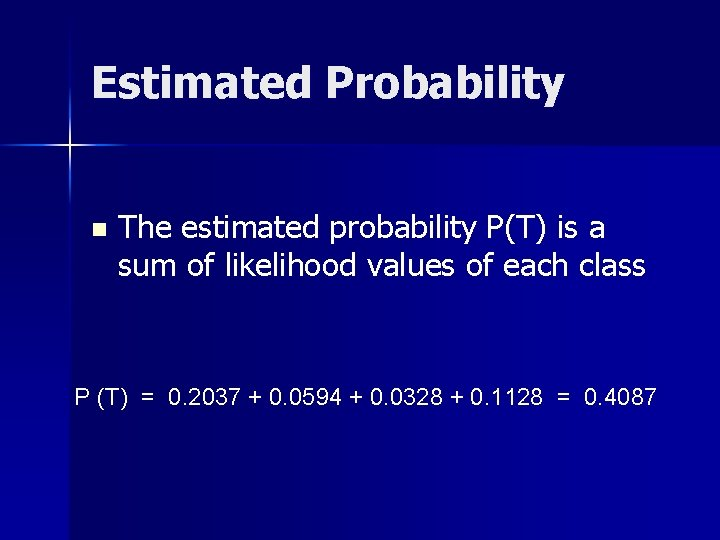Estimated Probability n The estimated probability P(T) is a sum of likelihood values of each class P (T) = 0. 2037 + 0. 0594 + 0. 0328 + 0. 1128 = 0. 4087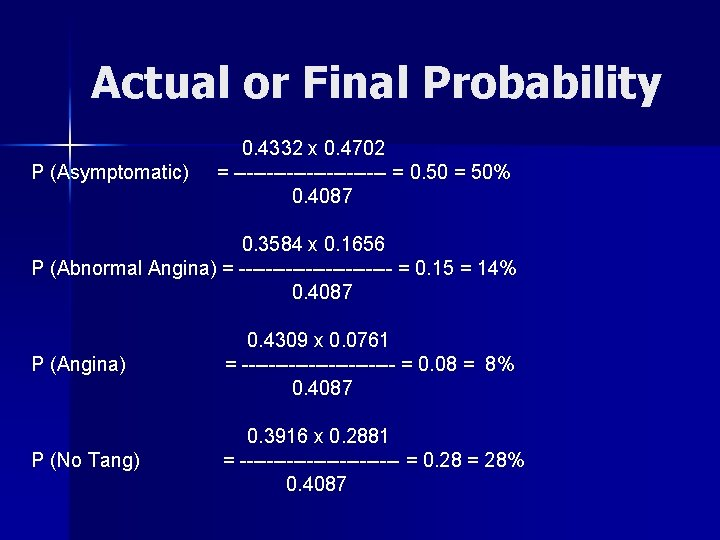Actual or Final Probability P (Asymptomatic) 0. 4332 x 0. 4702 = ------------ = 0. 50 = 50% 0. 4087 0. 3584 x 0. 1656 P (Abnormal Angina) = ------------ = 0. 15 = 14% 0. 4087 P (Angina) 0. 4309 x 0. 0761 = ------------ = 0. 08 = 8% 0. 4087 P (No Tang) 0. 3916 x 0. 2881 = ------------ = 0. 28 = 28% 0. 4087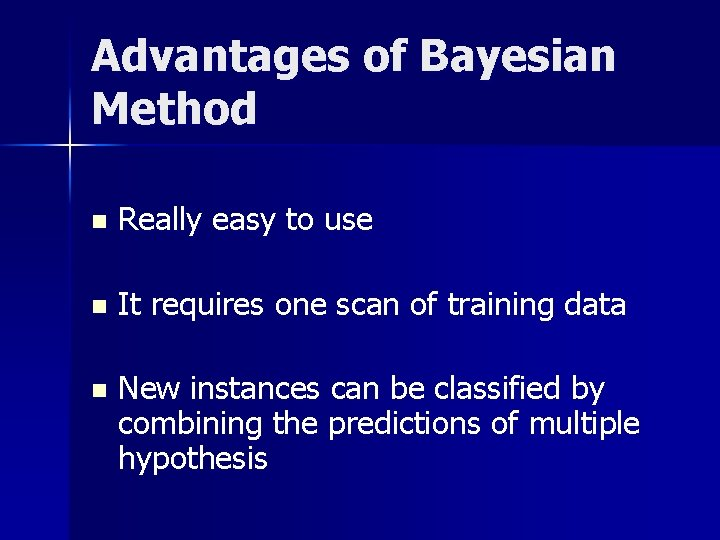Advantages of Bayesian Method n Really easy to use n It requires one scan of training data n New instances can be classified by combining the predictions of multiple hypothesis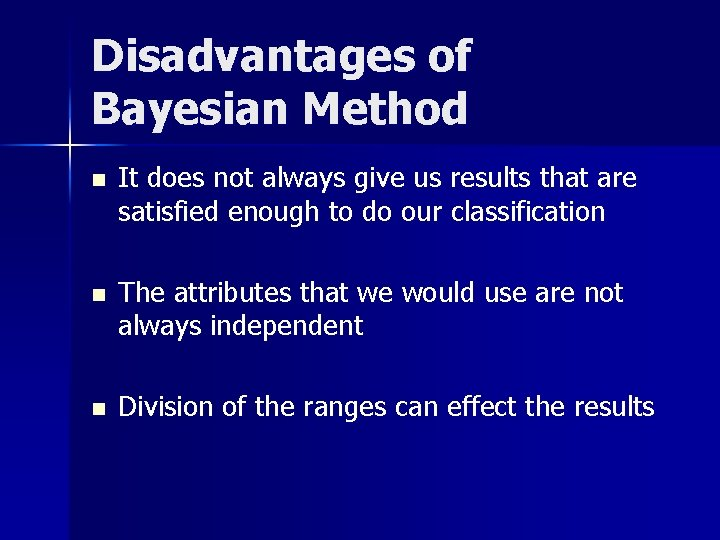Disadvantages of Bayesian Method n It does not always give us results that are satisfied enough to do our classification n The attributes that we would use are not always independent n Division of the ranges can effect the results